2021 NBA Defensive Player of the Year Odds
+300
3 to 1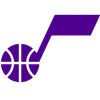Rudy Gobert
18.2% implied probability

+500
5 to 1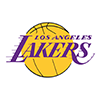Anthony Davis
12.2% implied probability

+750
7.5 to 1Myles Turner
8.6% implied probability

+850
8.5 to 1Giannis Antetokounmpo
7.7% implied probability

+1000
10 to 1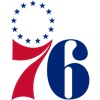Joel Embiid
6.6% implied probability

+1400
14 to 1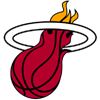Bam Adebayo
4.9% implied probability

No changes have been recorded yet.
+1500
15 to 1Ben Simmons
4.6% implied probability

+2000
20 to 1Draymond Green
3.5% implied probability

No changes have been recorded yet.
+2200
22 to 1Matisse Thybulle
3.2% implied probability

+2800
28 to 1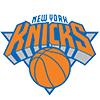Mitchell Robinson
2.5% implied probability

+3000
30 to 1Jrue Holiday
2.4% implied probability

+3500
35 to 1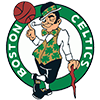Robert Williams
2.0% implied probability

+4000
40 to 1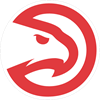Clint Capela
1.8% implied probability

+4000
40 to 1Deandre Ayton
1.8% implied probability

No changes have been recorded yet.
+5000
50 to 1Jimmy Butler
1.4% implied probability

No changes have been recorded yet.
+5000
50 to 1Jarrett Allen
1.4% implied probability

No changes have been recorded yet.
+5000
50 to 1Paul George
1.4% implied probability

+5500
55 to 1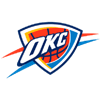Luguentz Dort
1.3% implied probability

No changes have been recorded yet.
+5500
55 to 1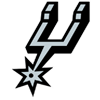Dejounte Murray
1.3% implied probability

+7000
70 to 1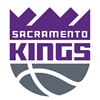Davion Mitchell
1.0% implied probability

No changes have been recorded yet.
+7000
70 to 1Jonathan Isaac
1.0% implied probability

+7000
70 to 1Marcus Smart
1.0% implied probability

+7500
75 to 1Dillon Brooks
1.0% implied probability

No changes have been recorded yet.
+8000
80 to 1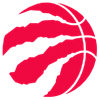OG Anunoby
0.9% implied probability

No changes have been recorded yet.
+8000
80 to 1Jaren Jackson
0.9% implied probability

No changes have been recorded yet.
+9000
90 to 1Karl-Anthony Towns
0.8% implied probability

+10000
100 to 1Royce O'Neale
0.7% implied probability

No changes have been recorded yet.
+10000
100 to 1Chris Boucher
0.7% implied probability

No changes have been recorded yet.
+10000
100 to 1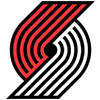Robert Covington
0.7% implied probability

No changes have been recorded yet.
+10000
100 to 1Nerlens Noel
0.7% implied probability

+10000
100 to 1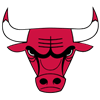Patrick Williams
0.7% implied probability

No changes have been recorded yet.
+10000
100 to 1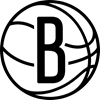Kevin Durant
0.7% implied probability

+12000
120 to 1Brook Lopez
0.6% implied probability

No changes have been recorded yet.
+12000
120 to 1P.J. Tucker
0.6% implied probability

+13000
130 to 1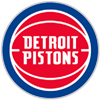Jerami Grant
0.6% implied probability

No changes have been recorded yet.
+15000
150 to 1Julius Randle
0.5% implied probability

No changes have been recorded yet.